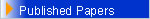Book EditorMathematics – Space Technology , Greece ,1980 Mathematical Analysis , Teubner–Texte zur Mathematik ,Vol. 79 , 1985 Mixed type Equations, Teubner–Texte zur Mathematik , Vol. 90 , 1986 Functional Analysis, Approximation Theory and Numerical Analysis, World Scientific, 340 pp,1994 Geometry, Analysis and Mechanics, World Scientific, 388 pp,1995 Advances in Equations and Inequalities , Hadronic Press , USA , 1999 Mathematical Equations and Inequalities , Vol. I , Greece ,1997 Mathematical Equations and Inequalities , Vol. II , Greece ,1999 Functional Equations, Integral Equations, Differential Equations and Applications, Intern. J. Appl. Math. & Stat., Vol. (1-6 Issues) dedicated to: the tri-centennial birthday anniversary of the Great L. P. EULER, 2007   BOOKS [In English] Editor : John Michael Rassias FUNCTIONAL ANALYSIS, APPROXIMATION THEORY AND NUMERICAL ANALYSIS Wold Scientific Publishing Co. Pte. Ltd, Singapore 1994, 1-325GEOMETRY, ANALYSIS AND MECHANICS Wold Scientific Publishing Co. Pte. Ltd, Singapore 1994, 1-376